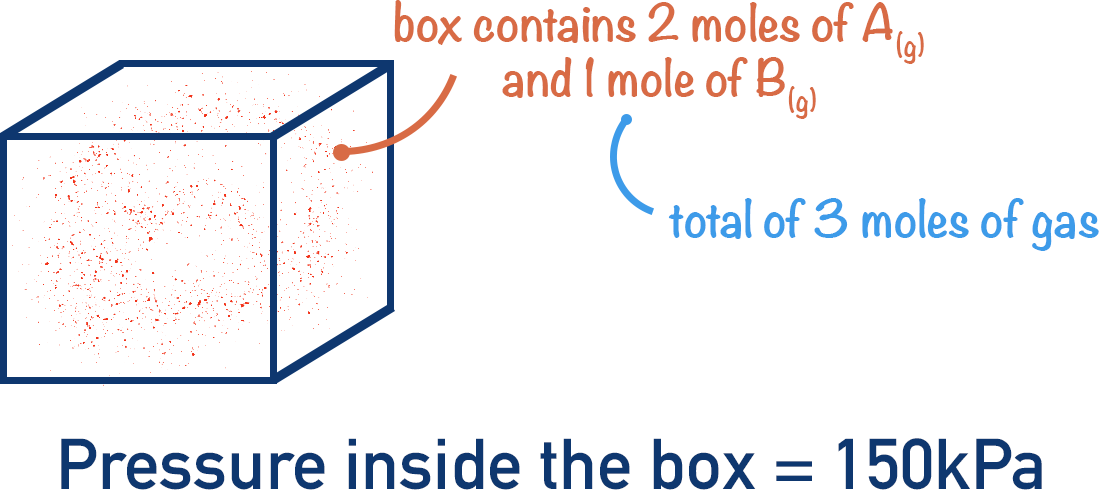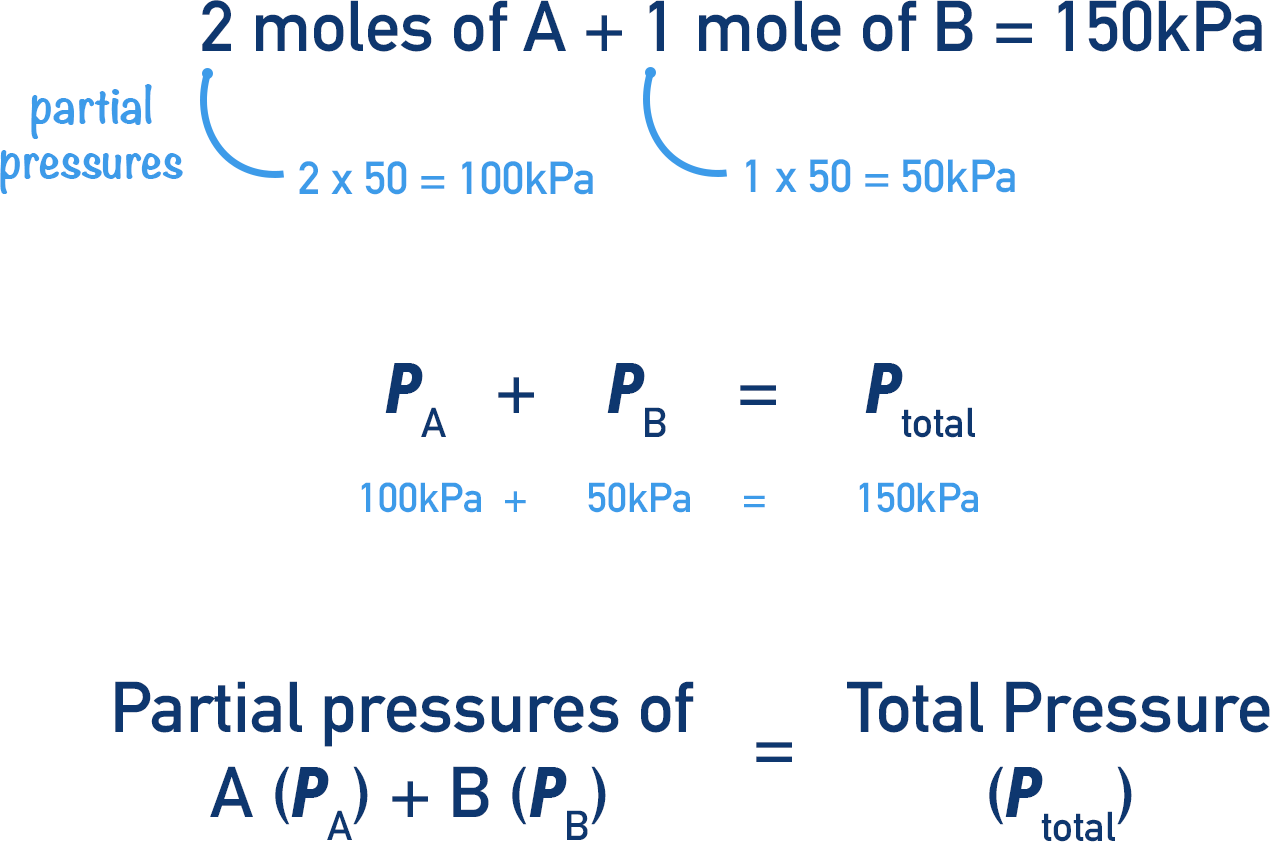Video Tutorial Mole Fractions and Partial Pressures

Quick Notes Moles Fractions and Partial Pressures

• How much pressure one type of gas contributes to the total pressure of a gaseous system is called its partial pressure.
• All partial pressures of gases in a system add up to give the total pressure of the system.
• The mole fraction of a type of gas describes how many moles of that gas there are compared to all other gases in the system.
• The total pressure of a gaseous system is directly related to the number of moles of all gases in the system.
• 1 mole of all gases are assumed to occupy the same volume in space (24 000cm3 at room temperature and pressure).

Full Notes Mole Fractions and Partial Pressures

If an equilibrium mixture is made up of just gaseous substances, then the total pressure of the ‘system’ at equilibrium is directly related to the number of moles of each substance.

Remember the ideal gas assumption: we assume that 1 mole of any gas occupies the same volume as 1 mole of any other gas. For example, 1 mole of hydrogen gas, (H2), is said to occupy the same volume as 1 mole of ethane (CH3CH3). Even though individual gas molecules are a different size, the impact of the size of a molecule is negligible compared to the volume it occupies in space. At room temperature and pressure, 1 mole of a gas is assumed to occupy 24dm3 (24 000cm3). See Ideal Gases.

Imagine a system made of 1 mole of A(g) and 1 mole of B(g), with a total pressure of 100kPa. We know there are 2 moles of gas in total, and A + B are contributing equal amounts of pressure to the system, as there are the same number of moles of each.

So, each gas is contributing 50kPa of pressure. This contribution is called the partial pressure of each gas.

A partial pressure is the contribution that a gas makes towards the total pressure of a system.

Imagine a sealed box that contains 2 moles of A(g) and 1 mole of B(g), with a total pressure of 150kPa.If three moles of gas cause a pressure of 150kPa, then one mole’s worth of gases must be responsible for 50kPa of pressure.There are two moles of A and one mole of B in the box. The partial pressure of A would be 100kPa (2 x 50kPa) and the partial pressure of B would be 50kPa (1 x 50kPa).This shows that the number of moles of a particular gas compared to the total number of moles of all gases is linked to its partial pressure. This ‘comparison’ is called its mole fraction.

A mole fraction is just a way of representing how much of a particular gas there is in a system compared to all gases present. Mole fractions are usually shown as decimals or fractions.

The partial pressure of a gas can then be calculated, by multiplying its mole fraction by the partial pressure of the system.

For example, air is made up of 79% nitrogen, 20% oxygen and 1% ‘other gases’. (Note, percentages are very approximate!) Assuming the pressure is 100 kPa.

Mole fraction of nitrogen = 79 /100

Mole fraction of oxygen = 20 /100 = 1/5

Mole fraction of ‘other gases’ = 1/100

Partial pressure of nitrogen = 79/100 x 100 kPa

Partial Pressure of oxygen = 20/100 x 100 kPa

Partial Pressure of ‘other gases’= 1 kPa